• What income distribution is "fair"?
• Sketch a histogram that represents what you believe to be the income distribution in the United States
• Include your guesses for the mean and median income

Fair Income

• Sketch a second histogram representing what you think should be the income distribution in the United States
• Include the mean and median income, and describe the shape, center, and spread of this histogram
• Give justification for what you think income distribution should look like

Data

• What data would help us understand the situation?
• What limitations might we have in obtaining this information?

Let's look at the data!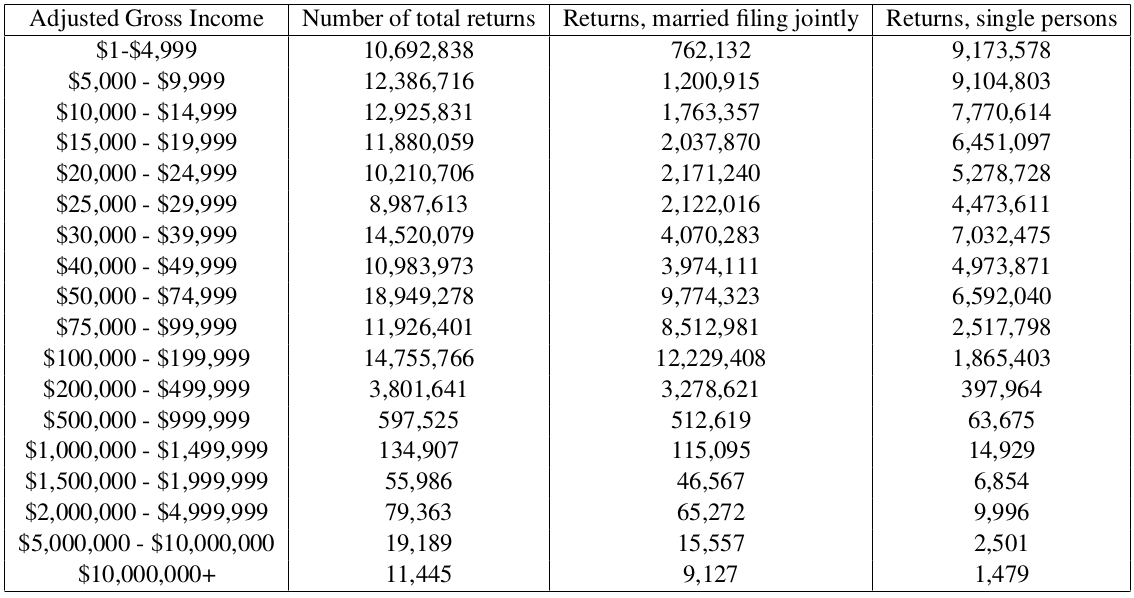• How might we want to represent this data?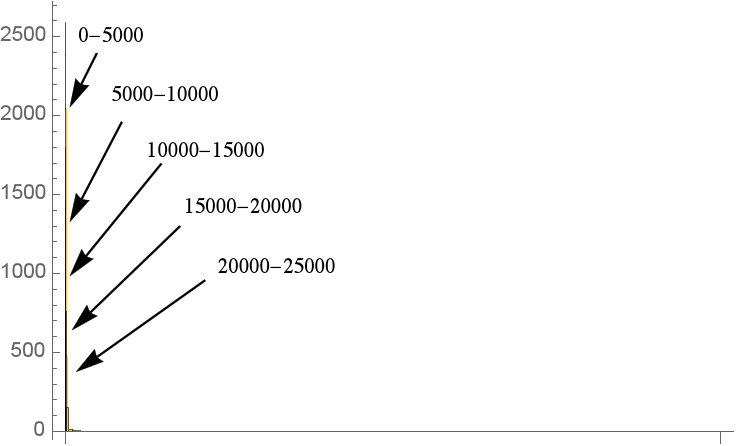1

Low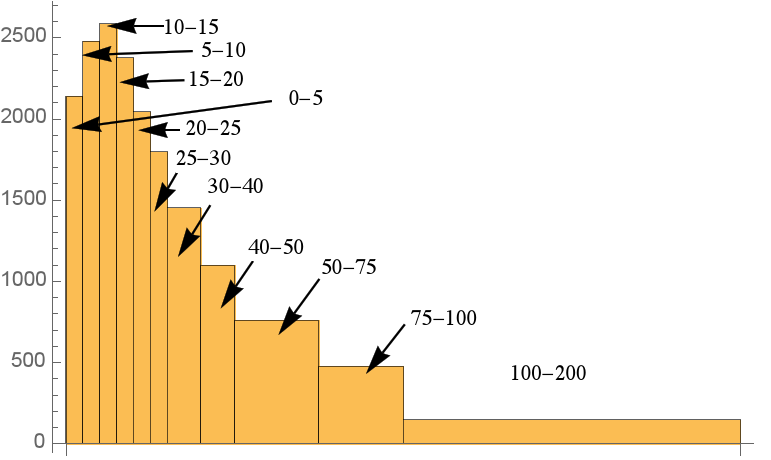2

High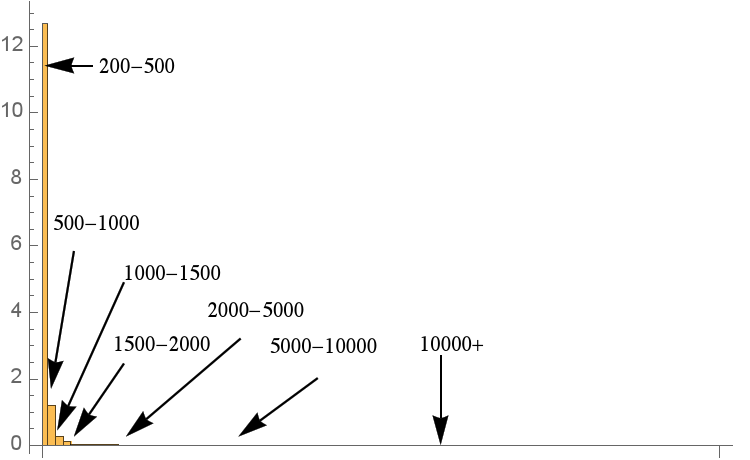3

Together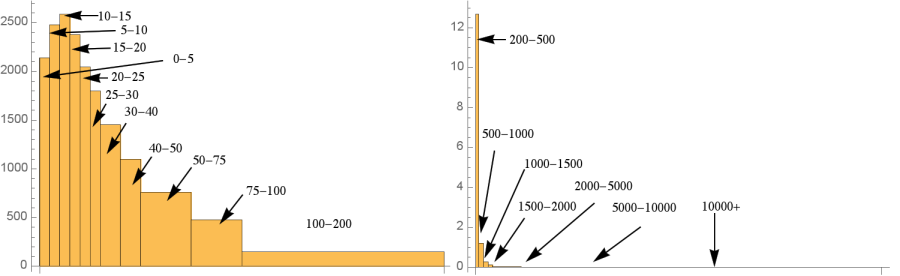4

• Notice that the bin corresponding to the greatest incomes does not have an upper limit.
• What might we choose as an upper limit? How could we justify this?

Analyzing the data

• Estimate the mean and median incomes. How do they compare, and why?
• What assumptions did you need to make to estimate the mean and median?
• Are these reasonable assumptions?

Interpreting

• What do these histograms tell us about income inequality in the U. S.?
• What surprises you about income inequality in the U. S.?
• How do we deal with the uppermost income bin being unbounded?
• How does using the lower, midpoint, or upper points of each bin interval affect the mean and median incomes?
• How does data presented in bins affect our calculating the mean and median of the data?
• Does it make histograms easier/ harder to create?
• What, if anything, do we gain (and what do we lose) from the presentation of data in this form versus the raw data?
• Who gets to choose the bin sizes?

Interpreting

• Median U. S. household income is $51324, and mean U. S. household income is$70909.
• Why might these be higher than the AGI data that we have?
• What might we hypothesize about the income distribution of households? (more skewed, less skewed, and why)